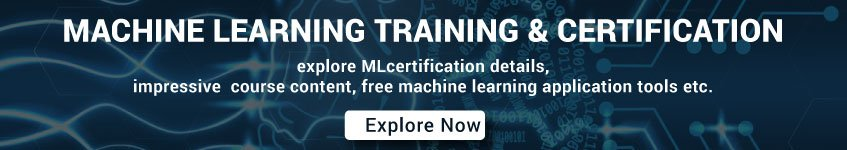# Machine Learning Interview Questions and Answers Set 10

91. What is the bias-variance decomposition of classification error in the ensemble method?

The expected error of a learning algorithm can be decomposed into bias and variance. A bias term measures how closely the average classifier produced by the learning algorithm matches the target function.  The variance term measures how much the learning algorithm’s prediction fluctuates for different training sets.

92. What is an Incremental Learning algorithm in the ensemble?

The incremental learning method is the ability of an algorithm to learn from new data that may be available after the classifier has already been generated from the already available dataset.

93. What are PCA, KPCA, and ICA used for?

PCA (Principal Components Analysis), KPCA ( Kernel-based Principal Component Analysis) and ICA ( Independent Component Analysis) are important feature extraction techniques used for dimensionality reduction.

94. What is dimension reduction in Machine Learning?

In Machine Learning and statistics, dimension reduction is the process of reducing the number of random variables under considerations and can be divided into feature selection and feature extraction

95. What are support vector machines?

Support vector machines are supervised learning algorithms used for classification and regression analysis.

96. What are the components of relational evaluation techniques?

The important components of relational evaluation techniques are

a)      Data Acquisition

b)      Ground Truth Acquisition

c)       Cross-Validation Technique

d)      Query Type

e)      Scoring Metric

f)       Significance Test

97. What is ensemble learning?

To solve a particular computational program, multiple models such as classifiers or experts are strategically generated and combined. This process is known as ensemble learning.

98. Why ensemble learning is used?

Ensemble learning is used to improve the classification, prediction, function approximation etc of a model.

99. When to use ensemble learning?

Ensemble learning is used when you build component classifiers that are more accurate and independent from each other.

100. What are the two paradigms of ensemble methods?

The two paradigms of ensemble methods are

a)      Sequential ensemble methods

b)      Parallel ensemble methods